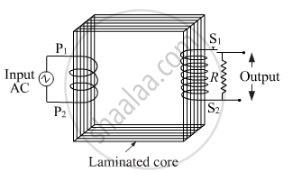# Draw a Necessary Arrangement for Winding of Primary and Secondary Coils in a Step-up Transformer - Physics

Draw a necessary arrangement for winding of primary and secondary coils in a step-up transformer. State its underlying principle and derive the relation between the primary and secondary voltages in terms of number of primary and secondary turns. Mention the two basic assumptions used in obtaining the above relation.

Draw a labelled diagram of a step-up transformer. Obtain the ratio of secondary to primary voltage in terms of number of turn and currents in the two coils.

#### SolutionPrinciple: It works on the principle of mutual induction. Whenever magnetic flux linked with coil changes, an emf is induced in the neighbouring coil.

Working: When an alternating emf is supplied to the primary coil then magnetic flux linked with the secondary coil charges. As a result, an induced emf is induced in the secondary coil. Voltage at the primary and the secondary coils depends on the number of turns. Magnetic flux that is linked with the primary coil is also linked with the secondary coil. The induced emf in each turn (Eturn) of the secondary coil is equal to that of the primary coil.

Let EP be the alternating emf applied to primary coil and np be the number of turns in it. If ϕ be the electric flux associated with it, then

E_p=−n_p (dϕ)/(dt)     .....(1)

where

(dϕ)/(dt)=Rate of change in flux across primary coil

Let Es be the emf across the secondary coil and ns be the no of turns in it. Then,

E_s=−n_s (dϕ)/(dt)     .....(2)

Dividing (2) by (1),

E_s/E_p=n_s/n_p=k

For step up transformer, k > 1

ES > EP

For step down transformer, k < 1

ES < EP

For ideal transformer. we assume that

1. The resistance of the primary and secondary windings are negligible.
2. The energy loss due to magnetic hysterisis in the iron core is negligible.

Concept: Inductance - Mutual Inductance
Is there an error in this question or solution?
2014-2015 (March) Patna Set 2

Share